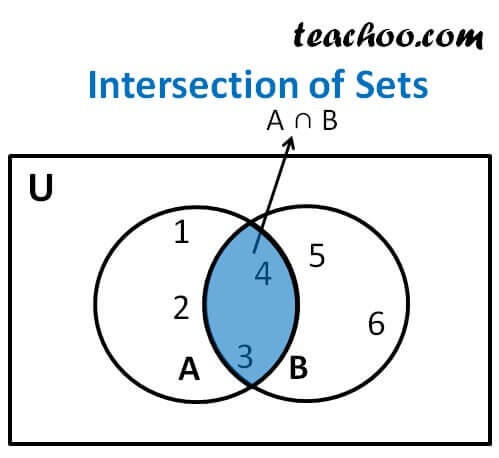Intersection of Sets

Chapter 1 Class 11 Sets
Concept wise

Intersection of sets A & B has all the elements which are common to  set A and set B

It is represented by symbol ∩

Let A = {1, 2, 3, 4 } , B = { 3, 4 , 5, 6}

A ∩ B = {3, 4}The blue region is A ∩ B

## Properties of Intersection

1. A ∩ B = B ∩ A (Commutative law).
2. (A  ∩ B) ∩ C = A ∩ (B ∩ C) (Associative law).
3. ∅ ∩ A = ∅, U ∩ A = A (Law of ∅ and U).
4. A ∩ A = A (Idempotent law)
5. A ∩ (B ∪ C) = (A ∩ B) ∪ (A ∩ C) (Distributive law) i. e., ∩ distributes over ∪
or we can also write it as
A ∪ (B ∩ C) = (A ∪ B) ∩ (A ∪ C)

Let us discuss these laws

Let us take sets

Let A = {1, 2, 3, 4} , B = {3, 4, 5, 6}, C = {6, 7, 8}

and Universal set = U = {1, 2, 3, 4, 5, 6, 7, 8, 9, 10}

### A ∩ B = B ∩ A (Commutative law).

A B = {1, 2, 3, 4} {3, 4, 5, 6} = {3, 4}

B ∩ A = {3, 4, 5, 6} {1, 2, 3, 4} = {3, 4}

∴ A ∩ B = B ∩ A

### (A  ∩ B) ∩ C = A ∩ (B ∩ C) (Associative law).

A B = {3, 4}

(A  ∩ B ) ∩ C = {3, 4} ∩ {6, 7, 8} = {} = ∅

B ∩ C = {3, 4, 5, 6} ∩ {6, 7, 8} = {6}

A ∩ (B ∩ C) = {1, 2, 3, 4} ∩ {6} = {} = ∅

∴ (A  ∩ B) ∩ C = A ∩ (B ∩ C)

### ∅ ∩ A = ∅, U ∩ A = A (Law of ∅ and U).

In intersection, we have all elements which are common

#### ∅ ∩ A = ∅

Since ∅ has no elements, there will be no common element between ∅ and A

∴ Intersection of ∅ and A will be ∅

∅ ∩ A = {} ∩ {1, 2, 3, 4} = {}

∴ ∅ ∩ A = ∅

#### U ∩ A = A

Since U has all the elements, the common elements between U and A will be all the elements of set A

∴ Intersection of U and A will be A

U ∩ A = { 1 , 2, 3, 4 , 5, 6, 7, 8, 9, 10} ∩ { 1, 2, 3, 4 }

U ∩ A = {1, 2, 3, 4} = A

∴ U ∩ A = A

### A ∩ A = A (Idempotent law)

A ∩ A = {1, 2, 3, 4} ∩ {1, 2, 3, 4}

A ∩ A = {1, 2, 3, 4} = A

∴ A ∩ A = A

### A ∩ (B ∪ C) = ( A ∩ B) ∪ (A ∩ C) (Distributive law) i . e., ∩ distributes over ∪

B ∪ C = {3, 4, 5, 6} ∪ {6, 7, 8} = {3, 4, 5, 6, 7, 8}

A ∩ (B ∪ C) = {1, 2, 3, 4 } ∩ { 3, 4, 5, 6, 7, 8} = {3, 4}

A B = {1, 2, 3, 4} {3, 4, 5, 6} = {3, 4}

A C = {1, 2, 3, 4} {6, 7, 8} = {} = ∅

(A ∩ B) ∪ (A ∩ C) = {3, 4} ∪ ∅ = {3, 4}

∴ A ∩ (B ∪ C) = (A ∩ B) ∪ (A ∩ C)

Let's also prove

## A ∪ (B ∩ C) = (A ∪ B) ∩ (A ∪ C) (Distributive Law)

B ∩ C = {3, 4, 5, 6 } ∩ { 6 , 7, 8} = {6}

A ∪ (B ∩ C) = {1, 2, 3, 4} ∪ {6} = {1, 2, 3, 4, 6}

A ∪ B = {1, 2, 3, 4} ∪ {3, 4, 5, 6} = {1, 2, 3, 4, 5, 6}

A ∪ C = {1, 2, 3, 4} ∪ {6, 7, 8} = {1, 2, 3, 4, 6, 7, 8}

(A ∪ B) (A ∪ C) = {1, 2, 3, 4, 5, 6} {1, 2, 3, 4, 6, 7, 8} = {1, 2, 3, 4, 6}

∴ A ∪ (B ∩ C) = (A ∪ B) ∩ (A ∪ C)

Learn in your speed, with individual attention - Teachoo Maths 1-on-1 Class